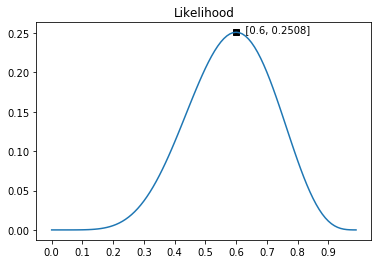# 最大似然估计：从概率角度理解线性回归的优化目标

## 概率和似然#

### 概率#

$P(6正4反 \ |\ \theta=0.5)=C_{10}^{6}\times 0.5^6 \times (1-0.5)^4 \approx 0.2051 \\ P(6正4反 \ |\ \theta=0.6)=C_{10}^{6}\times 0.6^6 \times (1-0.6)^4 \approx 0.2508 \\ P(6正4反 \ |\ \theta=0.9)=C_{10}^{6}\times 0.9^6 \times (1-0.9)^4 \approx 0.0112$

### 似然#

$L(\theta ; 6正4反)=C_{10}^{6}\times \theta^6 \times (1-\theta)^4 \\$“6正4反”的似然函数

$L(\theta ; \mathbf{X}) = P_1(\theta ; X_1) \times P_2(\theta ; X_2) ... \times P_n(\theta ; X_n) = \prod P_i(\theta; X_i)$

## 最大似然估计#

### 线性回归的最大似然估计#

$y^{(i)} = \epsilon^{(i)} + \sum_{j=1}^n w_jx_{j}^{(i)} =\epsilon^{(i)} + \boldsymbol{w}^\top \boldsymbol{x}^{(i)}$

$P(x ; \mu, \sigma) = \frac{1}{\sqrt{2 \pi \sigma^2}}\exp{({-\frac{(x - \mu)^2}{2 \sigma^2}})}$

$P(\epsilon^{(i)}) = \frac{1}{\sqrt{2 \pi \sigma^2}}\exp{({-\frac{(\epsilon^{(i)})^2}{2 \sigma^2}})}$

$P(y^{(i)}|\boldsymbol{x}^{(i)}; \boldsymbol{w}) = \frac{1}{\sqrt{2 \pi \sigma^2}}\exp{({-\frac{(y^{(i)} - \boldsymbol{w}^\top \boldsymbol{x}^{(i)})^2}{2 \sigma^2}})}$

$L(\boldsymbol{w}) = L(\boldsymbol{w}; \boldsymbol{X}, \boldsymbol{y}) = \prod_{i=1}^{m}P(y^{(i)}|\boldsymbol{x}^{(i)}; \boldsymbol{w})$

$L(\boldsymbol{w}) = \prod_{i=1}^{m} \frac{1}{\sqrt{2 \pi \sigma^2}}\exp{({-\frac{(y^{(i)} - \boldsymbol{w}^\top \boldsymbol{x}^{(i)})^2}{2 \sigma^2}})}$

\begin{aligned} \ell(\boldsymbol{w}) &= \log\ L(\boldsymbol{w}) \\ & = \log \ \prod_{i=1}^{m} \frac{1}{\sqrt{2 \pi \sigma^2}}\exp{({-\frac{(y^{(i)} - \boldsymbol{w}^\top \boldsymbol{x}^{(i)})^2}{2 \sigma^2}})} \\ &= \sum_{i=1}^{m}\log[\frac{1}{\sqrt{2 \pi \sigma^2}}\cdot\exp{({-\frac{(y^{(i)} - \boldsymbol{w}^\top \boldsymbol{x}^{(i)})^2}{2 \sigma^2}})}] \\ &= \sum_{i=1}^{m}\log[\frac{1}{\sqrt{2 \pi \sigma^2}}] + \sum_{i=1}^{m}\log[\exp{({-\frac{(y^{(i)} - \boldsymbol{w}^\top \boldsymbol{x}^{(i)})^2}{2 \sigma^2}})}] \\ &= m\log{\frac{1}{\sqrt{2 \pi \sigma^2}}} - \frac{1}{2 \sigma^2}\sum_{i=1}^{m}(y^{(i)} - \boldsymbol{w}^\top \boldsymbol{x^{(i)}})^2 \end{aligned}

$\log (ab) = \log(a) + \log(b) \\ \log_e (\exp(a)) = a$

$\sum_{i=1}^{m}(y^{(i)} - \boldsymbol{w}^\top \boldsymbol{x}^{(i)})^2$

1. 对目标函数求导，让导数为0，得到一个矩阵方程，矩阵方程的解即为模型的最优解
2. 使用梯度下降法，求模型的最优解。

## 总结#

1. 如何通俗地理解概率论中的「极大似然估计法」? - 马同学的回答 - 知乎 https://www.zhihu.com/question/24124998/answer/242682386
2. Andrew Ng：CS229 Lecture Notes